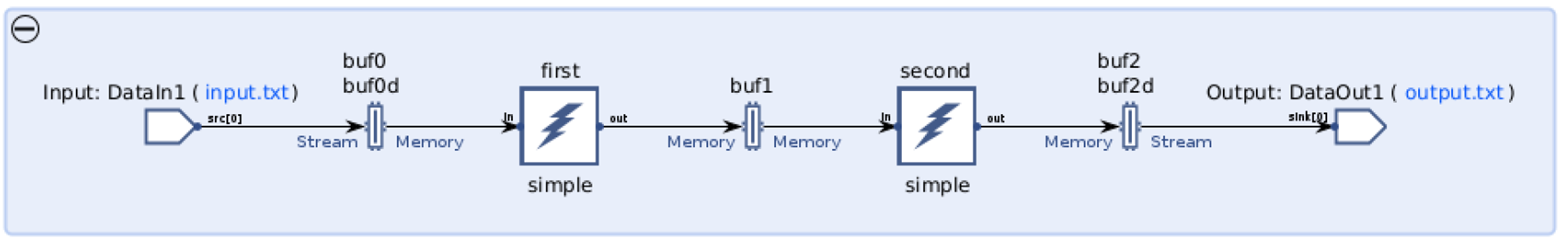# Creating a Data Flow Graph (Including Kernels) - 2021.2 English

## Versal ACAP AI Engine Programming Environment User Guide (UG1076)

Document ID
UG1076
Release Date
2021-12-17
Version
2021.2 English
This following process describes how to construct data flow graphs in C++.
1. Define your application graph class in a separate header file (for example `project.h`). First, add the Adaptive Data Flow (ADF) header (adf.h) and include the kernel function prototypes. The ADF library includes all the required constructs for defining and executing the graphs on AI Engines.
``````#include <adf.h>
#include "kernels.h"``````
2. Define your graph class by using the objects which are defined in the `adf` name space. All user graphs are derived from the class `graph`.
``````include <adf.h>
#include "kernels.h"

class simpleGraph : public graph {
private:
kernel first;
kernel second;
};``````

This is the beginning of a graph class definition that declares two kernels (`first` and `second`).

3. Add some top-level ports to the graph.
``````#include <adf.h>
#include "kernels.h"

class simpleGraph : public graph {
private:
kernel first;
kernel second;
public:
input_port in;
output_port out;

};``````
4. Use the `kernel::create` function to instantiate the `first` and `second` C++ kernel objects using the functionality of the C function `simple`.
``````#include <adf.h>
#include "kernels.h"

class simpleGraph : public graph {
private:
kernel first;
kernel second;
public:
input_port in;
output_port out;
simpleGraph() {
first = kernel::create(simple);
second = kernel::create(simple);
}
};``````
5. Add the connectivity information, which is equivalent to the nets in a data flow graph. In this description, ports are referenced by indices. The first input window or stream argument in the `simple` function is assigned index 0 in an array of input ports (`in`). Subsequent input arguments take ascending consecutive indices. The first output window or stream argument in the `simple` function is assigned index 0 in an array of output ports (`out`). Subsequent output arguments take ascending consecutive indices.
``````#include <adf.h>
#include "kernels.h"

class simpleGraph : public graph {
private:
kernel first;
kernel second;
public:
input_port in;
output_port out;

simpleGraph() {
first = kernel::create(simple);
second = kernel::create(simple);
connect< window<128> > net0 (in, first.in);
connect< window<128> > net1 (first.out, second.in);
connect< window<128> > net2 (second.out, out);
}
};``````This figure represents the graph connectivity specified in the previous graph code. Graph connectivity can be viewed when you open the compilation results in the Vitis analyzer. For more information, see Viewing Compilation Results in the Vitis Analyzer. As shown in the previous figure, the input port from the top level is connected into the input port of the first kernel, the output port of the first kernel is connected to the input port of the second kernel, and the output port of the second kernel is connected to the output exposed to the top level. The first kernel executes when 128 bytes of data (32 complex samples) are collected in a buffer from an external source. This is specified as a window parameter at the connection `net0`. Likewise, the second kernel executes when its input window has valid data being produced as the output of the first kernel expressed via connection `net1`. Finally, the output of the second kernel is connected to the top level output port as connection `net2`, specifying that upon termination the second kernel will produce 128 bytes of data.

6. Set the source file and tile usage for each of the kernels. The source file kernel.cc contains kernel first and kernel second source code. Then the ratio of the function run time compared to the cycle budget, known as the run-time ratio, and must be between `0` and `1`. The cycle budget is the number of instruction cycles a function can take to either consume data from its input (when dealing with a rate limited input data stream), or to produce a block of data on its output (when dealing with a rate limited output data stream). This cycle budget can be affected by changing the block sizes.
``````#include <adf.h>
#include "kernels.h"

class simpleGraph : public graph {
private:
kernel first;
kernel second;
public:
input_port in;
output_port out;
simpleGraph(){

first = kernel::create(simple);
second = kernel::create(simple);
connect< window<128> > net0 (in, first.in);
connect< window<128> > net1 (first.out, second.in);
connect< window<128> > net2 (second.out, out);

source(first) = "kernels.cc";
source(second) = "kernels.cc";

runtime<ratio>(first) = 0.1;
runtime<ratio>(second) = 0.1;

}
};``````
7. Define a top-level application file (for example project.cpp) that contains an instance of your graph class and connect the graph to a simulation platform to provide file input and output. In this example, these files are called input.txt and output.txt.
``````#include "project.h"

simpleGraph mygraph;
simulation::platform<1,1> platform("input.txt","output.txt");
connect<> net0(platform.src, mygraph.in);
connect<> net1(mygraph.out, platform.sink);

int main(void) {
mygraph.init();
ret=mygraph.run(<number_of_iterations>);
printf("Run failed\n");
return ret;
}
ret=mygraph.end();
Important: By default, the `mygraph.run()` option specifies a graph that runs forever. The AI Engine compiler generates code to execute the data flow graph in a perpetual `while` loop. To limit the execution of the graph for debugging and test, specify the `mygraph.run(<number_of_iterations>)` in the graph code. The specified number of iterations can be one or more.
ADF APIs have return enumerate type `return_code` to show the API running status.
The `main` program is the driver for the graph. It is used to load, execute, and terminate the graph. See Run-Time Graph Control API for more details.